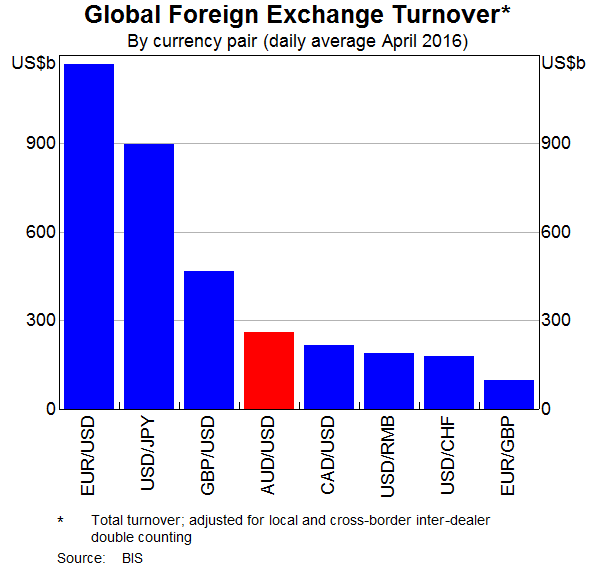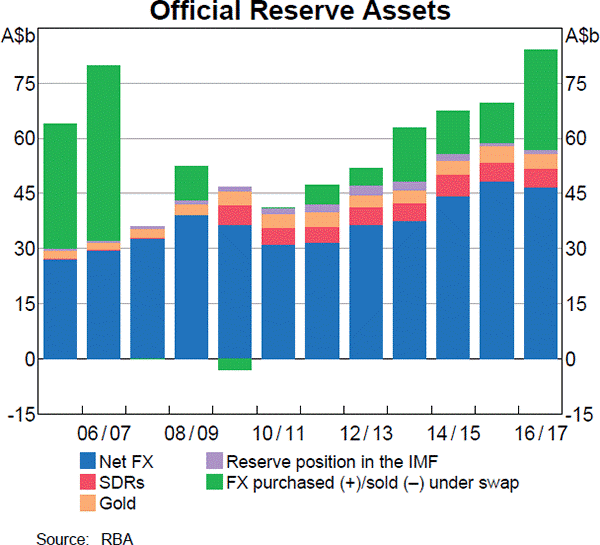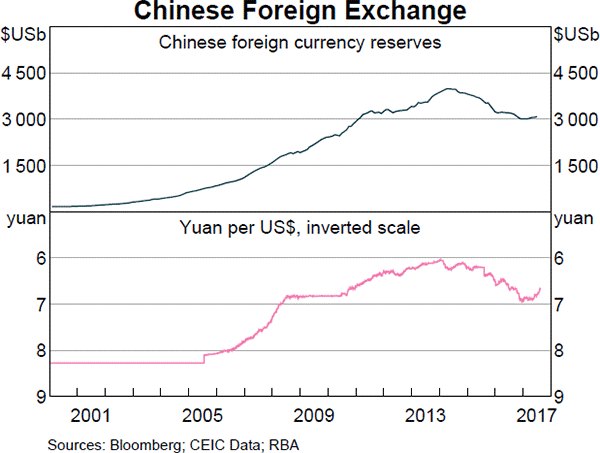# Fight Finance

#### CoursesTagsRandomAllRecentScores

According to the theory of the Capital Asset Pricing Model (CAPM), total risk can be broken into two components, systematic risk and idiosyncratic risk. Which of the following events would be considered a systematic, undiversifiable event according to the theory of the CAPM?

You just signed up for a 30 year fully amortising mortgage with monthly payments of $1,000 per month. The interest rate is 6% pa which is not expected to change. How much did you borrow? After 20 years, how much will be owing on the mortgage? The interest rate is still 6% and is not expected to change. A levered firm has a market value of assets of$10m. Its debt is all comprised of zero-coupon bonds which mature in one year and have a combined face value of $9.9m. Investors are risk-neutral and therefore all debt and equity holders demand the same required return of 10% pa. Therefore the current market capitalisation of debt $(D_0)$ is$9m and equity $(E_0)$ is $1m. A new project presents itself which requires an investment of$2m and will provide a:

• $6.6m cash flow with probability 0.5 in the good state of the world, and a • -$4.4m (notice the negative sign) cash flow with probability 0.5 in the bad state of the world.

The project can be funded using the company's excess cash, no debt or equity raisings are required.

What would be the new market capitalisation of equity $(E_\text{0, with project})$ if shareholders vote to proceed with the project, and therefore should shareholders proceed with the project?

An investor bought a 10 year 2.5% pa fixed coupon government bond priced at par. The face value is $100. Coupons are paid semi-annually and the next one is in 6 months. Six months later, just after the coupon at that time was paid, yields suddenly and unexpectedly fell to 2% pa. Note that all yields above are given as APR's compounding semi-annually. What was the bond investors' historical total return over that first 6 month period, given as an effective semi-annual rate? Which of the following FX quotes (current in October 2015) is given in American terms? Technical traders: Itau Unibanco is a major listed bank in Brazil with a market capitalisation of equity equal to BRL 85.744 billion, EPS of BRL 3.96 and 2.97 billion shares on issue. Banco Bradesco is another major bank with total earnings of BRL 8.77 billion and 2.52 billion shares on issue. Estimate Banco Bradesco's current share price using a price-earnings multiples approach assuming that Itau Unibanco is a comparable firm. Note that BRL is the Brazilian Real, their currency. Figures sourced from Google Finance on the market close of the BVMF on 24/7/15. Which form of production is included in the Gross Domestic Product (GDP) reported by the government statistics agency? The arithmetic average and standard deviation of returns on the ASX200 accumulation index over the 24 years from 31 Dec 1992 to 31 Dec 2016 were calculated as follows: $$\bar{r}_\text{yearly} = \dfrac{ \displaystyle\sum\limits_{t=1992}^{24}{\left( \ln⁡ \left( \dfrac{P_{t+1}}{P_t} \right) \right)} }{T} = \text{AALGDR} =0.0949=9.49\% \text{ pa}$$ $$\sigma_\text{yearly} = \dfrac{ \displaystyle\sum\limits_{t=1992}^{24}{\left( \left( \ln⁡ \left( \dfrac{P_{t+1}}{P_t} \right) - \bar{r}_\text{yearly} \right)^2 \right)} }{T} = \text{SDLGDR} = 0.1692=16.92\text{ pp pa}$$ Assume that the log gross discrete returns are normally distributed and that the above estimates are true population statistics, not sample statistics, so there is no standard error in the sample mean or standard deviation estimates. Also assume that the standardised normal Z-statistic corresponding to a one-tail probability of 2.5% is exactly -1.96. Which of the following statements is NOT correct? If you invested$1m today in the ASX200, then over the next 4 years:

Examine the below graphs. The first graph shows daily FX turnover in the world by both the public (government) and private sectors. The second graph 'Official Reserve Assets' shows the FX reserves of the Australian central bank, the RBA. The third graph's top panel shows the FX reserves of the Chinese central bank, the PBoC.Assume that the AUD and USD are priced at parity so 1 AUD = 1 USD.

Which of the following statements is NOT correct?